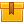# Fractions (A4303)

## Prerequisites

This lesson is designed to build on the skills presented in the second lesson of this program, Decimals.

## Description

This lesson teaches participants how to add, subtract, multiply, and divide fractions.

## Objectives

Identify a simple fraction and its components. Find equivalent fractions. Reduce fractions to lowest terms. Change improper fractions to mixed numbers. Change fractions to decimal equivalents. Estimate answers. Add fractions with common denominators. Add fractions with different denominators. Add fractions using pencil and paper. Add fractions using a calculator. Subtract fractions with common denominators. Subtract fractions by regrouping whole numbers. Subtract fractions by regrouping mixed numbers. Subtract fractions using a pencil and paper. Subtract fractions using a calculator. Multiply simple fractions. Multiply fractions and whole numbers. Multiply fractions and mixed numbers. Multiply fractions using a pencil and paper. Multiply fractions using a calculator. Divide simple fractions. Divide fractions and whole numbers. Divide fractions and mixed numbers. Divide fractions using pencil and paper. Divide fractions using a calculator.

## ContentThis lesson teaches participants how to add, subtract, multiply, and divide fractions.

•Fractions

0
0 Reviews